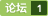### matlab解决非线性混合整数规划问题或者增广拉格朗日方法解决非线性规划问题 [问题点数：20分]1. <em>混合</em><em>整数</em><em>非线性</em><em>规划</em> <em>混合</em><em>整数</em><em>非线性</em><em>规划</em>，英文为Mixed-Integer NonLinear Programming (MINLP) ，指的是既包含<em>整数</em>约束，也包含<em>非线性</em>约束的优化<em>问题</em>。 2. 扩展割平面法 扩展割平面（Extended cutting plane，ECP）的思路是：使用线性约束来代替逐步代替<em>非线性</em>约束进行求解。 假设求解<em>问题</em>是： min f(x,y)f(x,...

<em>混合</em><em>整数</em><em>非线性</em><em>规划</em><em>matlab</em>代码，需要者请下吧~

Matlab求下列<em>整数</em>： %目标函数f,约束条件g function [f,g] = mengte(x) f = x(1)^2 + x(2)^2 + 3*x(3)^2 + 4*x(4)^2 + 2*x(5)^2 - 8*x(1) - 2*x(2) - 3*x(3) - x(4) - 2*x(5); g(1) = sum(x) - 400; g(2) = x(1) + 2*x(2) + 2*x

<em>非线性</em><em>整数</em><em>规划</em>的遗传算法Matlab程序

MINP混合整数非线性规划问题求解(MATLAB OPTI toolbox)
MINP(mixed integer nolinear programming)<em>问题</em>，是运筹学中的难点<em>问题</em>。常用的求解<em>方法</em>有分支定界法，割平面法。<em>混合</em><em>整数</em>的求解<em>问题</em>有一些稳定的求解器。比如ipsolve cplex, Matlab官方提供的GMATLAB使用智能仿生算法求解的。 本文介绍 OPTI toolbox 安装<em>方法</em>见：https://github.com/jonathancurrie/OP...

<em>非线性</em><em>整数</em><em>规划</em>是一个具有指数复杂度的NP<em>问题</em>，如果约束较为复杂，Matlab优化工具箱和一些优化软件比如lingo等，常常无法应用，即使能应用也不能给出一个较为令人满意的解。这时就需要针对<em>问题</em>设计专门
MATLAB求解线性规划（含整数规划和0-1规划问题

code function [f,g]=mengte(x); f=x(1)^2+x(2)^2+3*x(3)^2+4*x(4)^2+2*x(5)-8*x(1)-2*x(2)-3*x(3)... -x(4)-2*x(5); g(1)=sum(x)-400; g(2)=x(1)+2*x(2)+2*x(3)+x(4)+6*x(5)-800; g(3)=2*x(1)+x(2)+6*x(3)-200;...
matlab求解下面的线性规划整数规划
<em>matlab</em>求解下面的线性<em>规划</em>和<em>整数</em><em>规划</em>   本篇博客主要讲了用<em>matlab</em>实际求解<em>整数</em><em>规划</em><em>问题</em>，目前还没有时间去自己实现<em>整数</em><em>规划</em>算法，只能通过调用MATLAB的函数去实现。 题目 代码 第一小题 %线性<em>规划</em>代码 %author Canlong f=[-3,-2]; A=[2,3;1,0.5]; b=[14;4.5]; C=[]; d=[]; xm=[0;0]; xM=1e+10*[...
Matlab工具包混合整数规划
This directory contains the sources to the Mixed Integer Linear Program solver 'lp_solve' in gzip-ed tar format: lp_solve.tar.gz This is the supported version, which is written in strict ANSI C, meant for UNIX and contains porting information for VMS. The most current version is 3.2. Lp_solve 3.0 and newer are released under the Lesser GNU public License. Older versions live under a more restriction license, only non-commercial usage is free for them. Complaints about the programs should be sent to michel@magma-da.com. --------------------------------------------------------------------------- The currently latest version of lp_solve is 4.0. You can find a source kit (lp_solve_4.0.tar.gz), a pre-compiled Microsoft version (lp_solve_4.0_win32.zip) and a source kit that should compile under Visual C++ (lp_solve_4.0_vb.zip). lp_solve 4.0 is maintained by Peter Notebaert (peno@mailme.org). There are many older versions as well on this web site, if you feel the urge to play with them. ---------------------------------------------------------------------- There is a Java port of lp_solve 2.0 at: http://www.cs.wustl.edu/~javagrp/help/LinearProgramming.html ---------------------------------------------------------------------- There is a Perl interface available: start a search from http://search.cpan.org/ There are many other interfaces to lp_solve on the web; I advise a web search to find them.
bnb20求解混合整数非线性规划

matlab解线性规划问题
rn 未来属于终身学习者，唯有知识可以让我们免于平庸！rnrnrn 1rn&nbsp; 代码等资料可联系客服，凭购买截图获取（包括高清视频，适合电脑一族）；rnrnrn 2&nbsp;rn&nbsp;可咨询客服为你定制学习计划；rnrnrn 3&nbsp; &nbsp;1080P高清最新录制，音质清澈；rnrnrn 4&nbsp; &nbsp; 课程精炼，注重实战，注释详细，程序详解；rnrnrn rnrnrn rnrnrn rn
《Matlab在数学建模中的应用》笔记2-非线性规划&整数规划
《Matlab在数学建模中的应用》笔记2-<em>非线性</em><em>规划</em>&<em>整数</em><em>规划</em>

MATLAB规划问题——线性规划非线性规划
1.线性<em>规划</em> 求线性<em>规划</em><em>问题</em>的最优解有两种<em>方法</em>，一种<em>方法</em>是使用linprog命令，另一种是使用optimtool工具箱，下面分别介绍这两种<em>方法</em>. ①linprog命令 一般情况下，Linprog命令的参数形式为[x,fval] = linprog(f,A,b,Aeq,beq,lb,ub,x0)，下面分别介绍各参数的含义. [x,fval]返回值中x为最优解，fval为最优值. ...

MATLAB非线性规划函数
MATLAB<em>非线性</em><em>规划</em>函数 在 MATLAB\toolbox\optim中有两个M文件：constr．m和fminu．m，分别包含了用于约束优化<em>问题</em>和无约束优化<em>问题</em>的两组函数．下面介绍其中最有用的两个函数．
MATLAB求解非线性规划
MATLAB求解<em>非线性</em><em>规划</em>

Lingo
1、求解线性<em>规划</em> 输入如下： 结果：目标值=800， x1=250, x2=1002、求解二次<em>规划</em> 解： 3、求解： 解：model: sets: !集合部分; factory/A1..A3/:supply; !定义了一个factory集合，隐式定义集合中的成员为A1,A2,A3,集合中每个成员的属性为supply; market/B1..B4
matlab求解整数规划、0-1规划
<em>matlab</em>求解<em>整数</em><em>规划</em>、0-1<em>规划</em>R2014以前无法求解<em>整数</em><em>规划</em>，用bintprog求解0-1<em>规划</em>线性<em>规划</em>在2016版本中暂时还可用linprog求解求解0-1<em>规划</em>%p180task1 clear,clc; f=[10 12 15 11 16 13]; A=[40 60 80 50 90 70]; b=300; Aeq=[1 1 1 1 1 1]; beq=3; intcon=[1,2,3,4,5
Lingo与线性规划
Lingo与线性<em>规划</em> 代码如下 max =8*x1+6*x2; 9*x1+8*x2&amp;lt;=12; 7*x1+11*x2&amp;lt;=24; 9*x1+11*x2&amp;lt;=13; x1&amp;gt;=0; x2&amp;gt;=0;
LINGO解线性规划

matlab求解非线性规划

MATLAB非线性规划ppt
MATLAB<em>非线性</em><em>规划</em>课件，让初学者快速入门
matlab求解 非线性规划
<em>非线性</em><em>规划</em>min f(x)=(x1)^2+(x2)^2+(x3)^2+8 (x1)^2+x2+(x3)^2>0 x1+(x2)^2+(x3)^2=0; <em>matlab</em>求解编写函数1定义目标函数 function f=fun1(x); f=sum(x.^2)+8编写函数2定义<em>非线性</em>约束条件 function [g,f]=
matlab 非线性规划
<em>matlab</em> <em>非线性</em><em>规划</em>PPT，里面有关于<em>非线性</em><em>规划</em><em>问题</em>用<em>matlab</em>实现的几个函数讲解。

Lingo与极大化指派问题
Lingo与极大化指派<em>问题</em> 代码如下： model: !5个工人，5个工作的分配<em>问题</em>; sets: workers/w1..w5/; jobs/j1..j5/; links(workers,jobs): cost,volume; endsets !目标函数; min=@sum(links: cost*volume); !每个工人只能有一份...
lingo

matlab学习——非线性规划

<em>增广</em><em>拉格朗日</em>算法的<em>matlab</em>代码，同时有GUI窗口的演示，学习起来非常直观。
LINGO解非线性规划的数学软件
Lindo 和 Lingo 是美国 Lindo 系统公司开发的一套专门用于求解最优化<em>问题</em>的软件包。Lindo 用于求解线性<em>规划</em>和二次<em>规划</em><em>问题</em>,Lingo 除了具有 Lindo 的全部功能外,还可以用于求解<em>非线性</em><em>规划</em><em>问题</em>,也可以用于一些线性和<em>非线性</em>方程(组)的求解,等等。Lindo 和 Lingo 软件的最大特色在于可以允许优化模型中的决策变量是<em>整数</em>（即<em>整数</em><em>规划</em>）,而且执行速度很快。
lingo逻辑运算符

GAMS，优化调度，<em>混合</em><em>整数</em><em>规划</em>，gap，求解

yalmip + lpsolve + matlab 求解混合整数线性规划问题（MIP/MILP）

Algorithm之PrA：PrA之IP整数规划(包括0-1整数规划)算法经典案例剖析+Matlab编程实现
Algorithm之PrA：PrA之IP<em>整数</em><em>规划</em>算法经典案例剖析+Matlab编程实现 目录 分枝定界法 <em>整数</em><em>规划</em>例题 0-1<em>整数</em><em>规划</em>实例 分枝定界法 对有约束条件的最优化<em>问题</em>（其可行解为有限数）的所有可行解空间恰当地进行系统搜索，这就是分枝与定界内容。通常，把全部可行解空间反复地分割为越来越小的子集，称为分枝；并且对每个子集内的解集计算一个目...
matlab解决线性规划非线性规划，整形规划及代码

<em>非线性</em><em>规划</em>—分析与<em>方法</em> 阿佛里耳的大作 下载定有收获
MatLab建模学习笔记8——非线性规划问题求解

MATLAB非线性规划

matlab解0-1整数规划问题
-

如果目标函数或约束条件中包含<em>非线性</em>函数，就称这种<em>规划</em><em>问题</em>为<em>非线性</em><em>规划</em><em>问题</em>。一般说来，解<em>非线性</em><em>规划</em>要比解线性<em>规划</em><em>问题</em>困难得多。而且，也不像线性<em>规划</em>有单纯形法这一通用<em>方法</em>，<em>非线性</em><em>规划</em>目前还没有适于各种<em>问题</em>的一般算法，各个<em>方法</em>都有自己特定的适用范围。<em>非线性</em>规格的MATLAB解法 Matlab中的命令是[x,fval]=fmincon(fun,x0,A,b,Aeq,beq,lb,ub,nonlcon,o...
MATLAB数学建模(3)-非线性规划

matlab：无约束非线性规划函数
fminunc() fminsearch() %用于求解无约束<em>非线性</em><em>规划</em>的函数有：fminsearch和fminunc,用法介绍如下。 %fminsearch函数 %x=fminsearch(fun,x0) %x=fminsearch(fun,x0,options) %x=fminsearch(fun,x0,options,p1,p2,…) %[x,fval]=fminsearch(
MATLAB里实现整数规划
function =DividePlane(A,c,b,baseVector) %约束矩阵：A； %目标函数系数向量：c； %约束右端向量：b； %初始基向量：baseVector %目标函数取最小值
matlab用穷举法解决问题
<em>matlab</em>穷举法
Matlab——0-1整数规划
（1）第一个<em>问题</em>：指派<em>问题</em>的0-1<em>整数</em><em>规划</em><em>问题</em>，可以使用MATLAB，如果是其他的就不适用。 栗子： (1)\begin{bmatrix} 3 &amp;amp; 8 &amp;amp; 2 &amp;amp; 10 &amp;amp; 3 \\\\ 8 &amp;amp; 7 &amp;amp; 2 &amp;amp; 9 ...
Matlab——整数规划
<em>整数</em><em>规划</em>有很多解法，下面将一一列出： （1）分枝定界法——可求纯或<em>混合</em><em>整数</em>线性<em>规划</em>（隐枚举法） \quad（A）分枝。将可行解的空间反复地分割为越来越小的子集 \quad（B）定界。对每个子集内的解集计算一个目标下界 \quad（C）剪枝。若界限超出已知可行解集目标值的子集，不再分枝 （2）割平面法——可求纯或<em>混合</em><em>整数</em>线性<em>规划</em> （3）隐枚举法——求解“0-1”<em>规划</em>特殊情形 \quad（A）过滤隐枚...
matlab 源代码 整数规划
<em>matlab</em> 源代码 <em>整数</em><em>规划</em> 经典奉献
matlab求解整数规划
<em>matlab</em>求解<em>整数</em><em>规划</em>，可以自己修改，经检验可用

<em>整数</em><em>规划</em>，对于<em>整数</em>优化<em>问题</em>，采用MATLAB编程，比较方便实用

<em>matlab</em>代码适用于<em>解决</em>线性和<em>非线性</em><em>整数</em><em>规划</em>，<em>混合</em><em>整数</em><em>非线性</em><em>规划</em><em>问题</em>。
matlab的yalmip工具解决整数规划

<em>解决</em><em>整数</em><em>规划</em>中的0-1遗传算法代码 对于求0-1<em>规划</em>的朋友有一定帮助

&amp;lt;html&amp;gt; &amp;lt;head&amp;gt; &amp;lt;/head&amp;gt; &amp;lt;body&amp;gt; &amp;lt;script type=&quot;text/javascript&quot;&amp;gt; &amp;lt;!-- JavaScript代码 //--&amp;gt; &
matlab分支定界法解线性规划问题
<em>matlab</em>分支定界的实现，<em>整数</em><em>规划</em>，最优策略
Matlab 解非线性方程组2

MATLAB优化工具箱解线性规划（线性规划非线性规划

MATLAB 牛顿迭代法解非线性方程组

<em>整数</em><em>规划</em><em>整数</em><em>规划</em><em>整数</em><em>规划</em><em>整数</em><em>规划</em><em>整数</em><em>规划</em><em>整数</em><em>规划</em>
matlab学习——选址问题（线性规划非线性规划规划

VisualC.实效编程百例下载
VC++百例全套，VisualC.实效编程百例 相关下载链接：[url=//download.csdn.net/download/tmwanly/4784562?utm_source=bbsseo]//download.csdn.net/download/tmwanly/4784562?utm_source=bbsseo[/url]